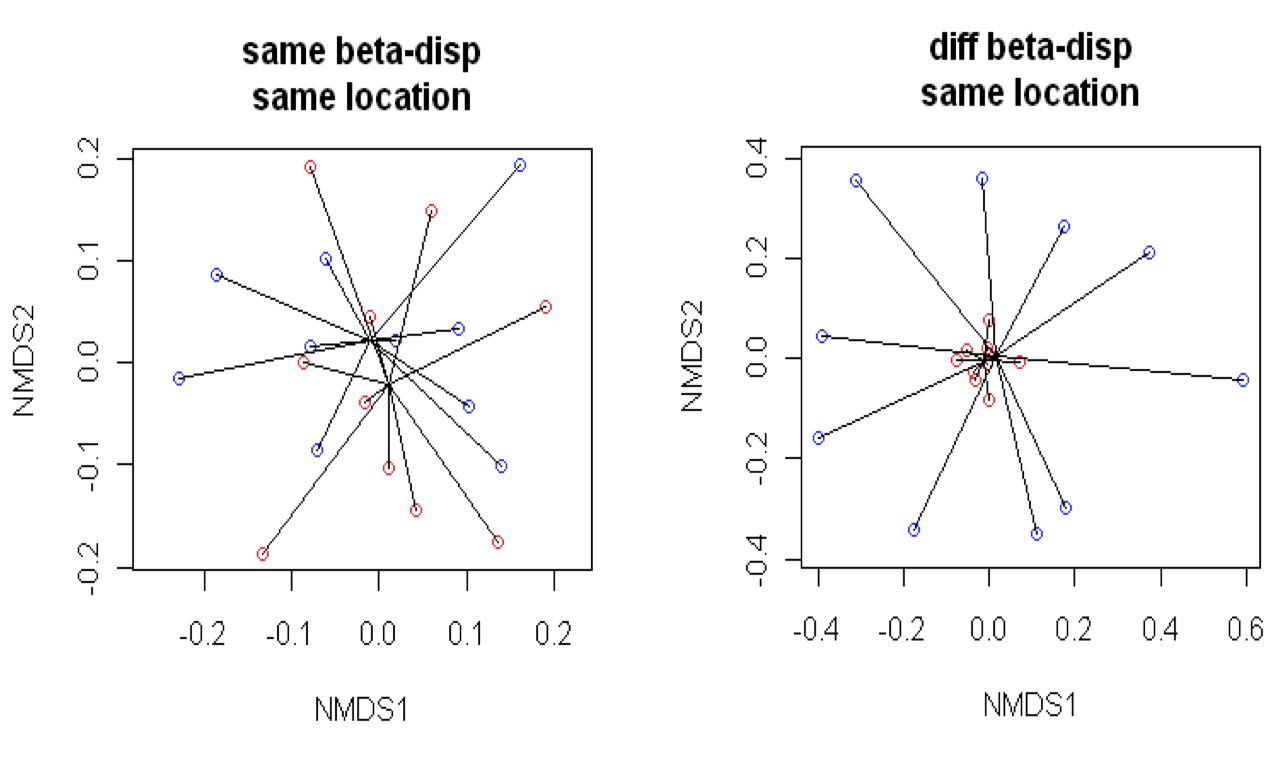## Multivariate analyses in R

By C Nell

#### Types of questions

Do groups differ in composition?
Does community structure vary among regions or over time?
Do environmental variables explain community patterns?
Which species are responsible for differences among groups?

Multivariate analysis of ecological communities with vegan

``````install.packages('vegan')
library(vegan) ##Community ecology: ordination, disversity & dissimilarities``````

#### Dataset

Bird abundances from 32 different plots (rows), 12 of which have 1 tree species (DIVERSITY = M) and 20 with 4 tree species (DIVERSITY = P).
Tree composition: there are a total of 6 possible tree species (treecomp), each signified with a letter A to F. Bird abundances are totalled according to their feeding guild (columns).

Get data from internet:

``````birds<-read.csv('https://raw.githubusercontent.com/collnell/lab-demo/master/bird_by_fg.csv')

``````setwd("/Users/colleennell/Dropbox/Projects/Mexico/R") #change to data folder
``````##   DIVERSITY PLOT CA FR GR HE IN NE OM
## 1         M    3  0  0  0  0  2  0  0
## 2         M    9  0  0  2  0  6  0  4
## 3         M   12  0  0  0  0  2  0  2
## 4         M   17  0  0  0  0  7  0  4
## 5         M   20  0  0  0  0  1  0  4
## 6         M   21  0  0  3  0 14  0  7``````
``````trees<-read.csv('tree_comp.csv')
``````##   PLOT comp A B C D E F row col
## 1    3    D 0 0 0 1 0 0   3   1
## 2    9    A 1 0 0 0 0 0   2   2
## 3   12    E 0 0 0 0 1 0   5   2
## 4   17    F 0 0 0 0 0 1   3   3
## 5   20    A 1 0 0 0 0 0   6   3
## 6   21    B 0 1 0 0 0 0   7   3``````

Questions: Is C. pentandara (B) associated with variation in bird species composition? Does feeding guild composition differ between monoculture and polyculture plots?

### MANOVA (Multivariate analysis of variance)

Parametric test for differences between independent groups for multiple continuous dependent variables. Like ANOVA for many response variables. Requires variables to be fewer than number of smaples.

Is C. pentandara (B) associated with variation in bird species composition? Or D & F (both Fabaceae)?

``````bird.matrix<-as.matrix(birds[,3:9])##response variables in a sample x species matrix
trees\$B<-as.factor(trees\$B)

bird.manova<-manova(bird.matrix~as.factor(B), data=trees) ##manova test
summary(bird.manova) ``````
``````##              Df  Pillai approx F num Df den Df  Pr(>F)
## as.factor(B)  1 0.39147   2.2056      7     24 0.07027 .
## Residuals    30
## ---
## Signif. codes:  0 '***' 0.001 '**' 0.01 '*' 0.05 '.' 0.1 ' ' 1``````

Show univariate results:

``summary.aov(bird.manova)``

#### Assumptions of MANOVA

1. Normal distribution
2. Linearity
3. Homogeneity of variances
4. Homogeneity of covariances

Problem: Most ecological data is overdispersed, has many 0’s or rare species, unequal sample sizes.
Solution: Dissimilarity coefficients, permutation tests

### PERMANOVA: Permutational multivariate analysis of variance

Non-paramentric, based on dissimilarities. Allows for partitioning of variability, similar to ANOVA, allowing for complex design (multiple factors, nested design, interactions, covariates). Uses permutation to compute F-statistic (pseudo-F).
Interactive app demonstrating permutation testsBased on Legendre & Anderson (1999, Ecological Monographs) and Anderson (2001, Austral Ecology).

Null hypothesis: Groups do not differ in spread or positioni n multivaraite space.

#### 1. Transform or standardize data

Use square root or proportions to minimize influence of most abundant groups.

``````bird.mat<-sqrt(bird.matrix)#square root transform
#bird.prop<-decostand(bird.matrix, method="total")``````

#### 2. Calculate ecological resemblance

Quantify pairwise compositional dissimilarity between sites based on species occurances.
- Bray-Curtis dissimilarity (abundance weighted)
- Jaccard (presence/absence)
- Gower’s (non-continuous variables)

Dissimilarity: 0 = sites are indentical, 1 = sites do not share any species

Create a dissimilarity matrix:

``bird.dist<-vegdist(bird.mat, method='bray')``

#### 3. perMANOVA

Do monoculture and polyculture plots differ in feeding guild composition?

``````set.seed(36) #reproducible results

bird.div<-adonis2(bird.dist~DIVERSITY, data=birds, permutations = 999, method="bray", strata="PLOT")
bird.div``````
``````## Permutation test for adonis under reduced model
## Terms added sequentially (first to last)
## Permutation: free
## Number of permutations: 999
##
## adonis2(formula = bird.dist ~ DIVERSITY, data = birds, permutations = 999, method = "bray", strata = "PLOT")
##           Df SumOfSqs      F Pr(>F)
## DIVERSITY  1  0.32857 4.1585  0.008 **
## Residual  30  2.37033
## ---
## Signif. codes:  0 '***' 0.001 '**' 0.01 '*' 0.05 '.' 0.1 ' ' 1``````

strata = ‘exchangeable units’ for permutation. Important for nested design.

#### 4. Multivariate dispersion

The average distance to group centroid. Used as a measure of multivariate beta diversity.``````dispersion<-betadisper(bird.dist, group=birds\$DIVERSITY)
permutest(dispersion)``````
``````##
## Permutation test for homogeneity of multivariate dispersions
## Permutation: free
## Number of permutations: 999
##
## Response: Distances
##           Df  Sum Sq   Mean Sq      F N.Perm Pr(>F)
## Groups     1 0.00369 0.0036924 0.2231    999  0.638
## Residuals 30 0.49659 0.0165530``````
``plot(dispersion, hull=FALSE, ellipse=TRUE) ##sd ellipse``